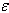SEARCH HOMEMath Central Quandaries & QueriesQuestion from moulipriya, a student: If G be a commutative group, and H be a proper subgroup of G. Then will H be always commutative?Moulipriya,

Suppose that H is a subgroup of G. Let * be the group operation on G andbe the group operation on H. Let a and b be elements of H. Since H is a subgroup of G you know that

• a * b = ab
• b * a = ba
• abH
• baH

If G is commutative is H commutative also?

PennyMath Central is supported by the University of Regina and The Pacific Institute for the Mathematical Sciences.